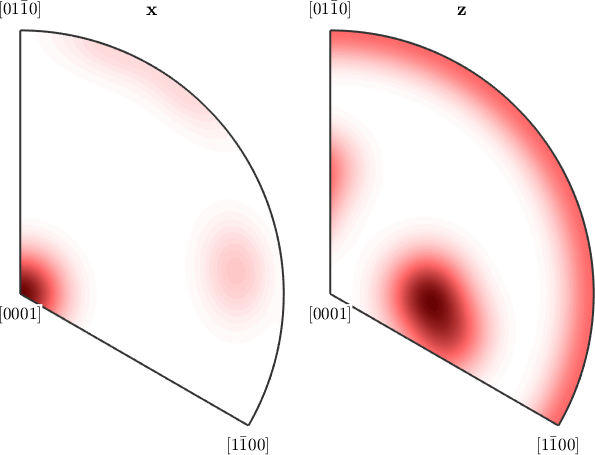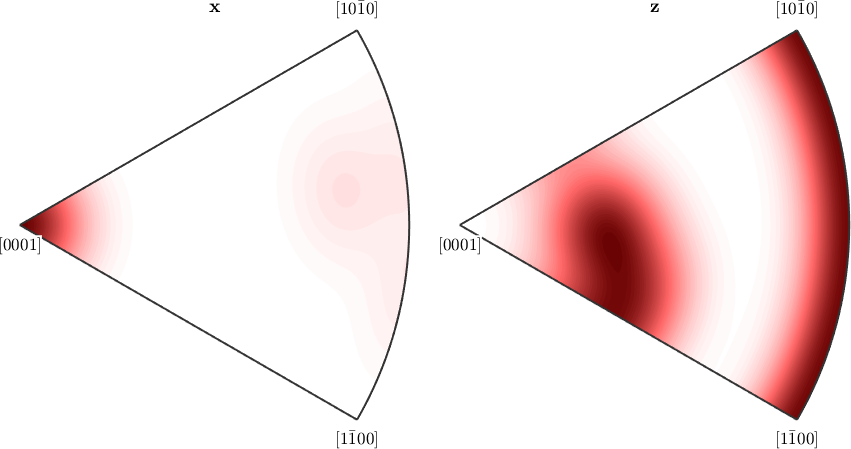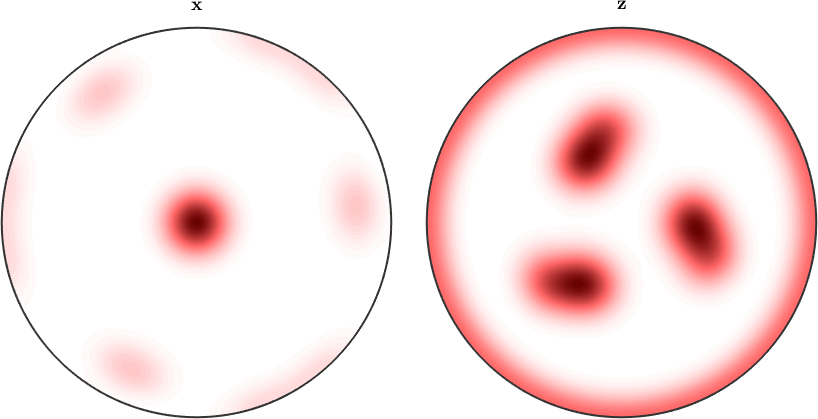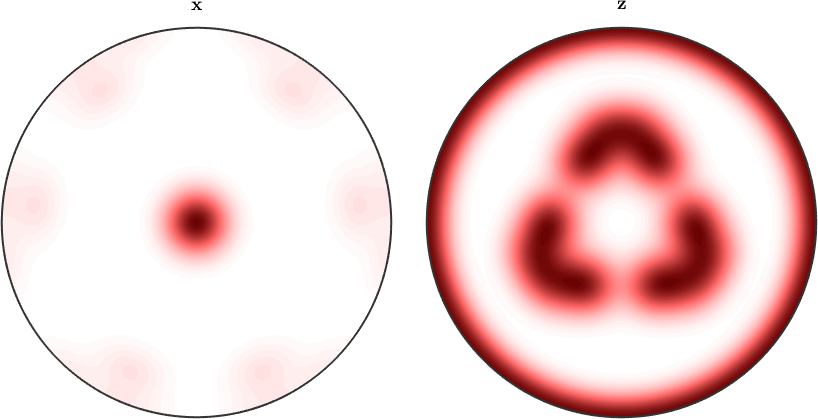Inverse Pole Figures edit page

## Theory

For an orientation distribution function (ODF) $$f \colon \mathrm{SO}(3) \to R$$ the inverse pole density function $$P_{\vec r}$$ with respect to a fixed specimen direction $$\vec r$$ is spherical function defined as the integral

$P_{\vec r}(\vec h) = \int_{g \vec h = \vec r} f(g) dg$

The pole density function $$P_{\vec r}(\vec h)$$ evaluated at a crystal direction $$\vec h$$ can be interpreted as the volume percentage of crystals with the crystal lattice planes $$\vec h$$ beeing normal to the specimen direction $$\vec r$$.

In order to illustrate the concept of inverse pole figures at an example lets us first define a model ODF to be plotted later on.

and lets switch to the LaboTex colormapImposing antipodal symmetry to the inverse pole figures halfes the fundamental regionBy default MTEX always plots only the fundamental region with respect to the crystal symmetry. In order to plot the complete inverse pole figure you have to use the option complete.This illustrates also more clearly the effect of the antipodal symmetryFinally, lets set back the default colormap.